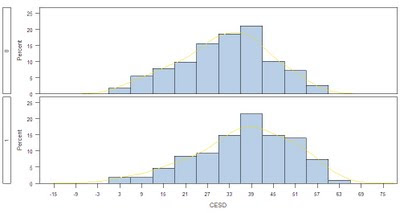## Monday, June 27, 2011

### Example 8.42: skewness and kurtosis and more moments (oh my!)While skewness and kurtosis are not as often calculated and reported as mean and standard deviation, they can be useful at times. Skewness is the 3rd moment around the mean, and characterizes whether the distribution is symmetric (skewness=0). Kurtosis is a function of the 4th central moment, and characterizes peakedness, where the normal distribution has a value of 3 and smaller values correspond to thinner tails (less peakedness).

Some packages (including SAS) subtract three from the kurtosis, so that the normal distribution has a kurtosis of 0 (this is sometimes called excess kurtosis.

R
`library(moments)library(lattice)ds = read.csv("http://www.math.smith.edu/r/data/help.csv")ds\$gender = ifelse(ds\$female==1, "female", "male")densityplot(~ cesd, data=ds, groups=gender, auto.key=TRUE)`

We see that the distribution of CESD scores is skewed with a long left tail, and appears somewhat less peaked than a normal distribution. This is confirmed by the actual statistics:
`> with(ds, tapply(cesd, gender, skewness))    female       male -0.4906171 -0.2464390 > with(ds, tapply(cesd, gender, kurtosis))    # kurtosis  female     male 2.748968 2.547061 > with(ds, tapply(cesd, gender, kurtosis))-3  # excess kurtosis    female       male -0.2510318 -0.4529394 `

SAS
SAS includes much detail on the moments and other statistics in the output from proc univariate. As usual, the quantity of output can be off-putting for new users and students. Here we extract the moments we need with the ODS system. We also generate kernel density estimates roughly analogous to the densityplot() results shown above.
`ods output moments = cesdmoments;proc univariate data="c:\book\help.sas7bdat";  class female;  var cesd;  histogram cesd / kernel;run;proc print data=cesdmoments;   where label1 = "Skewness";  var female label1 nvalue1 label2 nvalue2;run;`

With the result:
`Obs   FEMALE    Label1         nValue1    Label2         nValue2  4     0      Skewness      -0.247513   Kurtosis      -0.442010 10     1      Skewness      -0.497620   Kurtosis      -0.204928`

We note that the default is to produce unbiased (REML) estimates, rather than the biased method of moments estimator produced by the kurtosis() function (and that SAS presents the excess kurtosis).Nick Horton said...

A reader pointed out that the "e1071" package provides three different flavors for each measure. The corresponding documentation discusses some of the properties and which statistical software prefers which version. This is another option to consider to calculate these quantities.

Ken Kleinman said...

I probably also should have added that different estimates for these properties can be generated in SAS using the vardef option to the proc univariate statement. To get the results shown from the kurtosis() function in the moments package, use vardef=n.

efrique said...

Skewness is the 3rd moment around the mean, and characterizes whether the distribution is symmetric (skewness=0).

A couple of issues:

First, (Pearson) skewness is a standardized third central moment (third central moment divided by the cube of the standard deviation - it doesn't change when you change from meters to feet, even though the third central moment does).

Second, skewness = 0 does not imply symmetry - nonsymmetric counterexamples are easy to construct.

Indeed, symmetry doesn't necessarily imply zero third central moment (take the Cauchy distribution for an example).

So to say that third-moment based skewness characterizes symmetry is too strong.

http://ecstathy.blogspot.com/2008/06/not-fooling-ourselves-i-unmeasuring-of.html

efrique said...

(followup)
I realized just after clicking "post" that
I shouldn't have called it Pearson skewness (since there are a bunch of things that get called that), but should have said moment-based skewness (rather less ambiguous).

Ken Kleinman said...

Hi, Efrique--

Thanks for those clarifications. For what should be a straightforward topic, there's a lot of loose language used in discussing both skewness and kurtosis. Most likely we should use explicit formulae, rather than mere words, when precision about them seems important. And one hopes that an assertion of symmetry will be based on inspection of the pdf, not just the skewness, however calculated.